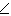# CAT Quantitative Aptitude: Previous year Solved Questions on Ratios

Find out the application Problems Based on Ratios that includes the problems that have appeared on Ratios in CAT exams across various years from 1999 to 2007.CAT Quantitative Aptitude Ratios

‘Ratios’ is one of the most expected topics in CAT exam every year. Questions from this concept are either directly or indirectly asked from the aspirants in quantitative aptitude section. These questions come in the form of mixtures and allegations as well. Aspirants preparing for the CAT and other important MBA entrance exams must not miss out on this topic as it carries significant weightage in the arithmetic section. Rather, other topics such as geometry and trigonometry also carry indirect application of Ratios in various questions.

Read on to find out various types of questions that might be asked in the exam from this topic. Here are various examples that will illustrate the forms in which questions on Ratios are often asked in the MBA entrance exams:

Problem 1 and Problem 2:

Sweetness of various items relative to Sucrose, whose sweetness is taken as 1 are shown in the table below.

Lactose 0.16
Maltose 0.32
Glucose 0.74
Sucrose 1.00
Fructose 1.70
Saccharin 675.00

Problem 1:

What is the minimum amount of Sucrose to be added to 1 gm. Saccharin to make the mixture at least 100 times sweeter than glucose?

 7

 8

 9

 100

Solution:

Sweetness 100 times that of glucose means 0.74x100 = 74

Sweetness at least 100 times of glucose means 74 or more.

Let the minimum amount of Sucrose that needs to be added to Saccharin be ‘x’Looking at the choices, options  fits the answer.

Since the question asks for minimum amount of Sucrose to be added to 1 gm. Saccharin, hence option  is correct.

Note: Options 2 & 3 are incorrect because we need to add minimum Sucrose to Saccharin to get a mixture at least 100 times sweeter than Glucose. We will achieve this by adding 7 gm. of Sucrose to 1 gm. Of Saccharin. You may double check the answer by substituting 7 in inequation (i). If the R.H.S of inequation (i) is greater than or equal to 74, then 7 gm. should be the right answer. In fact, in the exam time, one may form inequation (i) and then check the answers by going backwards using different options given in the question.

Problem 2:
Approximately how many times sweeter than Sucrose is the mixture consisting of Glucose, Sucrose, Fructose in the ratio 1: 2 : 3 ?

 1.3

 1

 0.6

 2.3

Solution:Hence, option  is correct.

Problem 3:

Ram has a box in which there are coins of denominations 50 paise, 25 paise, and 1 rupee. Total sum is Rs 210. Ratio of coins is . What is the number of 50 paisa coins?

 126

 200

 42

 44

Solution:

Value equation is

(0.50x3x) + (0.25x4x) + (1.00x2.5 x) = 210

Therefore x = 42

Therefore, the number of 50 paise coins is 42x3 = 126.

Hence, option  is correct.

Problem 4:
A jogging park has two identical circular tracks touching each other, and a rectangular track enclosing the two circles. The edges of the rectangles are tangential to the circles. Two friends, ‘A’ and ‘B’, start jogging simultaneously from the point where one of the circular tracks touches the smaller side of the rectangular track. ‘A’ jogs along the rectangular track, while ‘B’ jogs along the two circular tracks in a figure of eight. Approximately, how much faster than A does B have to run, so that they take the same time to return to their starting point?

 3.88%

 4.22%

 4.44%

  4.72%

Solution:

A B
Distance Travelled 12r 4πr
Speed 12r 4πr
Diving by 4r 3 π

(As the ratio of speed is the same as the ratio of distance travelled)

B has to run (π-3)/3x100 faster than A. Or B runs= 0.14/3x100=4.67 = 4.72% fsater than A.

Hence, option  is correct.

Problem 15:
In the following figure, the diameter of the circle is 3 cm. AB and MN are two diameters such that MN is perpendicular to AB. In addition, CG is perpendicular to AB such AE : EB that and DF is perpendicular to AB such that NL: LM = 1 : 2 the length of Dh is cm is2√2-1

 (2√2-1)/2

 (3√2-1)/2

 (2√2-1)/3

Solution:

AE: EB = 1:2; AE = 1; EO =  ; OB = 3/2 (radius);

Similarly LN = 1; OL = 1/2 ; OM = = 3/2 (radius)

Now, Join OD to form ‘Right Angled Triangle’ ODL. Applying Pythagoras Theorem in triangle ODL,

(LD)2 = (OD) 2- (OL)2

therefore (LD)2 = {3/2)2 - {1/2} 2

= 9/4-1/4 = 2 therefore LD = √2

Now, DH = LD – HL

√2-1/2 = {2√2-1/2}. Therefore, Option 2 is the correct choice

Problem 16:

Consider the triangle ABC shown in the following figure where BC=12 cm, DB =9cm, CD= 6cm, and  .What is the ratio of the perimeter of the triangle ADC to that of the triangle BDC?

 7/9

 8/9

 7/9

 5/9

Solution:

LetBCD =BAC =ø

∆ABC□∆CBD

AC/CD = AB/BC = BC/BD

AC/6 = 12/9                       [Using AC/CD = BC/BD]

AC = 8

(AD+9)/12= 12/9              [[Using AC/CD = AB/BC]

AB = AD +9 = 16